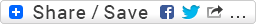## Graphics over code in Impromptu

Andrew Sorensen posted some code in the Impromptu mailing list showing how to add graphics (and various other things) on top of a code ‘image’. As usual I regret not having a whole free day to play with this, but just by messing around a bit with some parameters it’s easy to achieve some interesting effects…

Here’s the source code from Andrew:

``````
(gfx:start-live-video)

(define canvas (gfx:make-canvas 640 480))

(define gl (gl:make-opengl))
(gl:open-opengl gl '(0 0 640 480))

;; draw code behind a rotating cube
;; if you wanted you could now call gfx:start-movie-capture
;; and pass the gl context where canvas usually goes.

(define loop-gl
(lambda (beat)
(let* ((code (gfx:get-code-image))
(flipped (gfx:flip-image code))
(width (car (gfx:get-image-size code)))
(height (cdr (gfx:get-image-size code))))
(gl:clear gl (+ *gl:color-buffer-bit* *gl:depth-buffer-bit*))
(gl:window-pos gl 0 0 .99)
(gl:draw-pixels gl width height *gl:rgba* *gl:unsigned-byte* flipped)
(gl:translate gl 0 0 .5)
(gl:rotate gl (* beat 10) 1 1 1)
(gl:color gl 1 1 1 1)
(glut:wire-cube gl 0.5)
(gl:flush gl)
(gl:update-backing-image gl)
(objc:release (+ (now) 5000) code flipped)
(callback (*metro* (+ beat (* .5 1/6))) 'loop-gl (+ beat 1/6)))))

(loop-gl (*metro* 'get-beat 4))8

;; define an image to use as layer3

(define layer3 (gfx:make-image 640 480))

;; draw paths to layer3
(define draw-on-layer3
(lambda (beat)
(gfx:clear-image layer3)
(dotimes (i 10)
(gfx:path2image (gfx:make-circle (cosr 320 200 (/ i 100))
(sinr 240 200 (/ i 100))
20)
layer3
'() (list (/ i 5) 0 1 1)))
(callback (*metro* (+ beat (* .5 1/6))) 'draw-on-layer3 (+ beat 1/6))))

(draw-on-layer3 (*metro* 'get-beat 4))

;; draw video and layer3 transparently into the opengl bitmap
;; then render the opengl bitmap to the canvas
;;
;; You could easily replace
;; (gl:get-image-from-opengl gl) with (gfx:get-code-image)
;; if you didn't want to use any opengl

(define capture-code
(lambda (time)
(let ((video (gfx:get-live-frame))
(opengl (gl:get-image-from-opengl gl)))
(gfx:image2image video opengl .3)
(gfx:image2image layer3 opengl 1)
(gfx:draw-image time canvas opengl .9)
(objc:release (+ time 5000) opengl video))
(callback (+ time 2000) 'capture-code (+ time 5000))))

(capture-code (now))

;; start-movie-capture takes a canvas OR an opengl context
;; so you could call (gfx:start-movie-capture gl ...
;; as long as your gl code calls gl:update-backing-image

(gfx:start-movie-capture canvas "~/tmp/my.mov" #t)
;(gfx:stop-movie-capture canvas)

``````